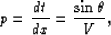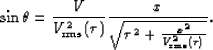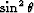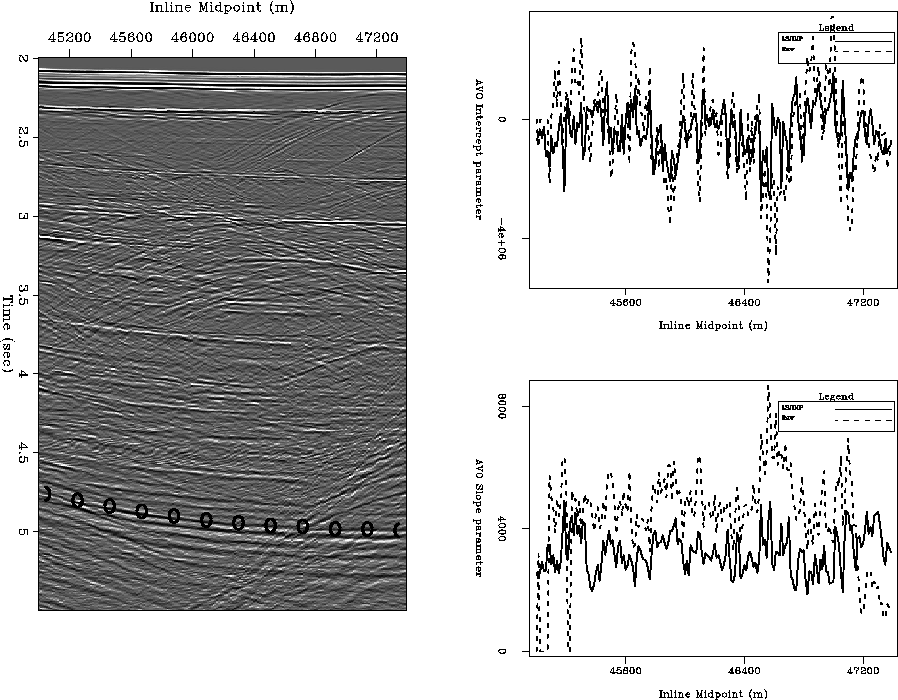Next: Conclusions \label>chapter:conclusions> Up: Results Previous: LSJIMP versus Radon Demultiple

## AVO Analysis Before and After LSJIMP

Amplitude-versus-offset or AVO analysis is perhaps the most commonly utilized direct hydrocarbon indicator in exploration reflection seismology today. The stacked results before and after LSJIMP on the Green Canyon 3-D dataset showed that stacking greatly attenuates multiples. However, from the prestack data, we saw that the multiples are prominent, and would surely inhibit signal processing techniques, like AVO analysis, if left intact. In this section, I illustrate how LSJIMP improves the AVO analysis of the Green Canyon 3-D data.

Shuey (1985) showed that in a 1-D earth, the measured reflection strength of an event at the surface is approximately linear with the square of its incidence angle, at angles less than 30 degrees. In a 1-D earth, the NMO equation gives an approximate relationship between offset and incidence angle. Claerbout (1995) defines the stepout'', p, as the spatial derivative of an event's traveltime curve:(37)
whereis the incidence angle and V is the velocity at the surface; in the marine case, simply water velocity. Stepout was earlier used to derive the Snell Resampling operator in Section. In a 1-D earth, the traveltime curve of a primary reflection is approximately given by the NMO equation (). Taking the derivative of equation () with respect to offset, then substituting into equation () gives the following expression for the sine of incidence angle as a function of offset:(38)
Reflection data as a function of offset may be mapped tovia equation (), at which point the AVO slope'' and intercept'' parameters may be estimated, usually via a linear least-squares fit to the data after resampling from offset to.

Figureillustrates the estimation of AVO slope and intercept parameters on a deep reflector in the Green Canyon 3-D data, before and after application of LSJIMP. The reflector, which is well under the multiples in the data, is denoted on the zero offset section with O'' symbols. The maximum amplitude in a small time window around the reflection were picked automatically, and make up the input data to the least-squares estimation.maxampl.gc3d.4-5
Figure 10
AVO parameter estimation for a deep reflector. Left: zero offset section, O'' symbols denote reflector of interest. Right, top: AVO intercept parameter before and after LSJIMP, as a function of midpoint. Right, bottom: AVO slope parameter before and after LSJIMP, as a function of midpoint.

We see that while the parameter estimates contain the same trends before and after LSJIMP, the LSJIMP result is more consistent and less noisy'' across midpoint. My implementation of LSJIMP works on a CMP-by-CMP basis, so the results shown in Figureare not smoothed across midpoint. The similarity across midpoint is an expression of the true lithology - lithology which LSJIMP better reveals.

Figureillustrates, as a function of midpoint, the small time windows taken around the deep reflector shown in Figure, before and after LSJIMP. The input data to an AVO parameter estimation are picked maximum amplitudes within the time window as a function of. Notice the significant increase in reflector clarity after LSJIMP. Also recall that the data residuals (e.g., in Figuresand) are quite small. Therefore, the cleaner reflection events after LSJIMP in Figureare not only better for AVO analysis - they also fit the recorded data in a quantitative fashion. LSJIMP is not an ad hoc post-processing technique.avogather.gc3d.4-5
Figure 11
Small time windows around the deep reflector shown in Figure, before and after LSJIMP. Individual panels along the vertical axis correspond to windows taken at different midpoint locations. Left: Data windows before LSJIMP. Right: Data windows after LSJIMP.Next: Conclusions \label>chapter:conclusions> Up: Results Previous: LSJIMP versus Radon Demultiple
Stanford Exploration Project
5/30/2004